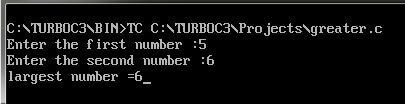UncleCoder.com

UncleCoder.com

Free programming examples and instructions

# C program to find largest among two numbers

## C program for how to find largest among two numbers

by Krishna

Posted on 27 Jul 2018 Category: C Views: 2321

C program to find the largest among two numbers

``````#include <stdio.h>
#include <conio.h>

void main() {
int a, b;
printf("Enter the first number :");
scanf("%d ", &a);
printf("Enter the second number :");
scanf("%d ", &b);

if(a > b)
{
printf("Largest number =%d", a);
}
else if (b > a)
{
printf("Largest number =%d", b);
}
else
{
printf("Both are Equal\n");
}

getch();
}``````

OUTPUT